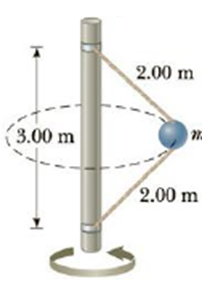Chapter 7, Problem 69AP

Chapter
Section
Textbook Problem

A 4.0-kg object is attached to a vertical rod by two strings as shown in Figure P7.69. The object rotates in a horizontal circle at constant speed 6.00 m/s. Find the tension in (a) the upper string and (b) the lower string.Figure P7.69

(a)

To determine
The tension in the upper string.

Explanation

Given info: Mass of the object (m) is 4.00 kg. The speed at which the object rotates (v) is 6.00 m/s.

Explanation:

The free body diagram is,

From the diagram,

(T1T2)cosθ=mg       (I)

(T1+T2)sinθ=mv2rsinθ       (II)

• v is the speed of the ball.
• r is the distance between the object and the center.
• g is the acceleration due to gravity.
• T1 and T2 are the tensions on the strings.

The distance between the object and the center is,

r=(2.00m)2(3.00m2)2=1.32m

The angle of inclination is,

θ=cos1[(3.00m2)2.00m]=cos1(0

(b)

To determine
The tension in the lower string.

Still sussing out bartleby?

Check out a sample textbook solution.

See a sample solution

The Solution to Your Study Problems

Bartleby provides explanations to thousands of textbook problems written by our experts, many with advanced degrees!

Get Started

Find more solutions based on key concepts